## SASA Viya (Explore & Visualise) - incorrect division answer

Hi, SAS Viya is giving me an incorrect answer for a simple division problem.

Referring to the List Table, there are 3 columns - Calculated Item 1, Calculated Item 2 & Calculated Item 3.

Calculated item 3 = Calculated Item 2 / Calculated Item 1) in percentage.

Using the 1st row as an example,

Calculated Item 1 = 290,397

Calculated Item 2 = 169,804.74

Calculated Item 3 = 38.50% (which is incorrect. Correct answer should be 58.47%).

I have tried changing the aggregation to Sum as well as Average but none works.

Appreciate if someone can tell me what went wrong.

Thank you.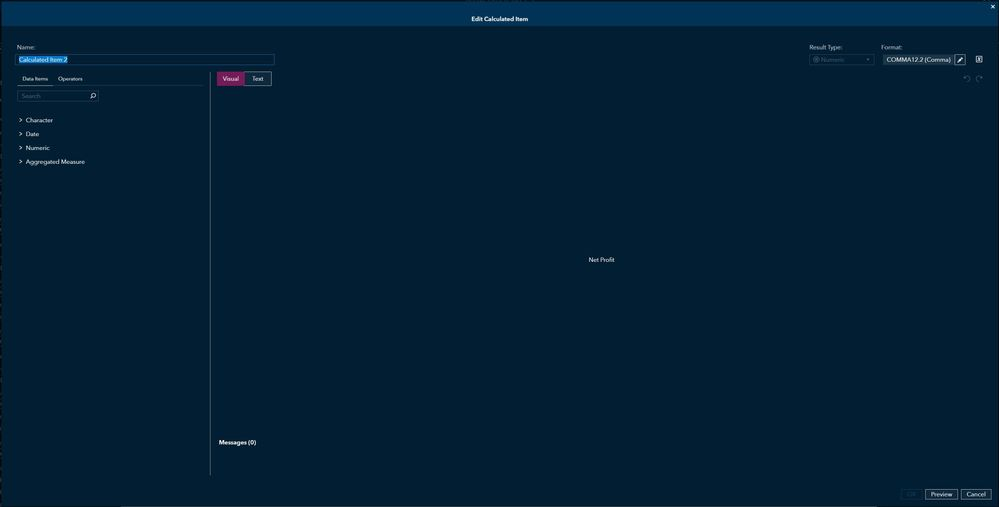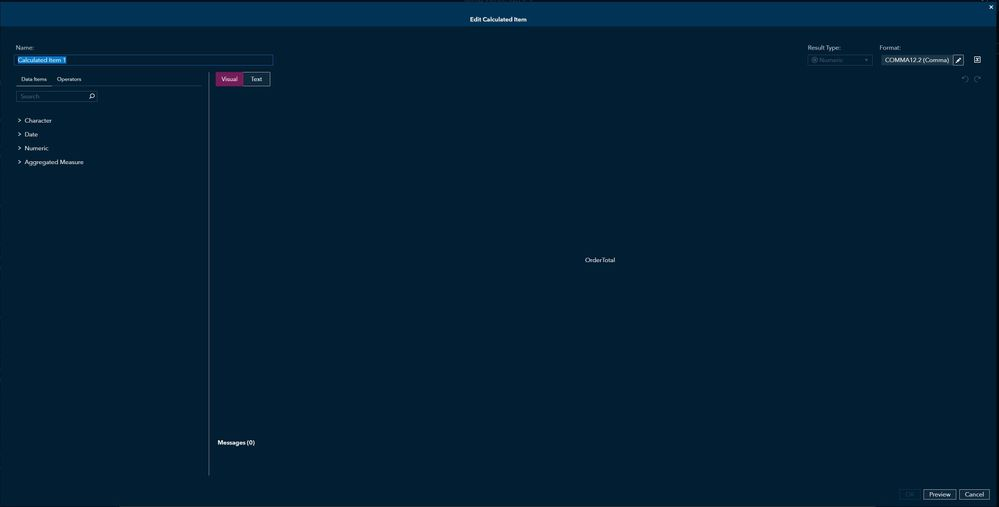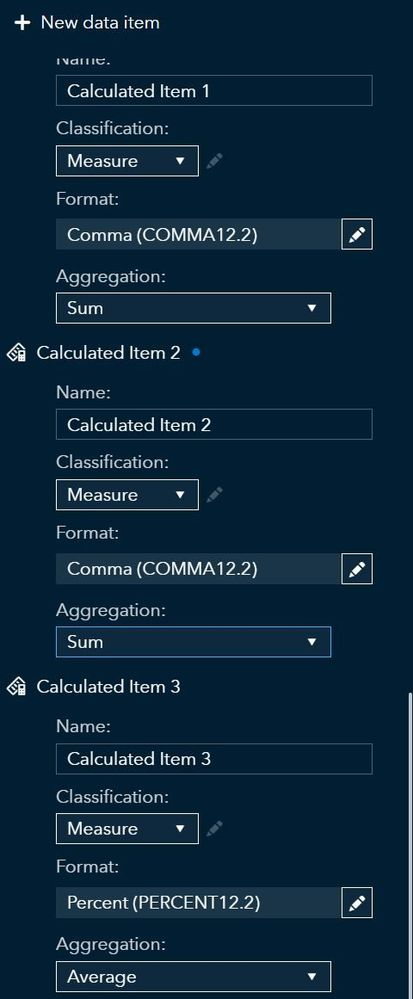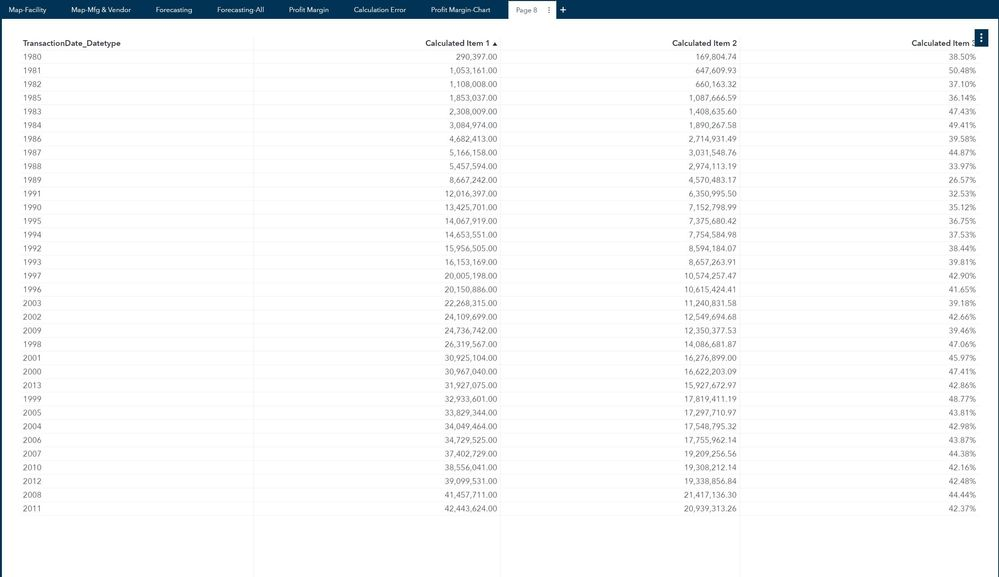7 REPLIES 7Sam_SAS
SAS Super FREQ

## Re: SASA Viya (Explore & Visualise) - incorrect division answer

Hello,

By default, all calculations are performed on a row-by-row basis (based on the rows in your data rather than the aggregated values displayed in your list table object.) If your table does not display detail data, then the numbers can appear not to match up because of the way they are aggregated.

Try changing your expression for item 3 to be like this:

`Sum [_ByGroup_] ('Calculated Item 2'n) / Sum [_ByGroup_] ('Calculated Item 1'n)`

I think this should work if the aggregations for all of the items match. When I was testing this I got REALLY confused because one of my measures had its aggregation as Count and the results were really bizarre! 😲

Let us know if that helps,

Sam

## Re: SASA Viya (Explore & Visualise) - incorrect division answer

Hi Sam,

Thank you so much for your help.

I tried recreating using your suggestion and it worked!

However the measures are now being classified as Aggregated Measure and cannot be use to carry out other analytics such as linear regression and forecasting.

Do you have any other suggestions on how I might be able to work around it?

The problem I encountered arises when I had to calculate the net profit( which is the calculated item 3) by using OrderTotal less Total Expenses which is made up of 4 cost items (Product, Marketing, Distribution & Sales).

I was able to calculate gross profit without using any aggregate measures; the only difference is that Gross Profit is calculated using OrderTotal Less Product Cost.

Do you have any idea why I was able to calculate gross profit and not net profit?

Regards,

KSSam_SAS
SAS Super FREQ

## Re: SASA Viya (Explore & Visualise) - incorrect division answer

Hi KS,

Is your Total Expenses a calculated item? Can you show what that expression looks like?

Thanks,
Sam

## Re: SASA Viya (Explore & Visualise) - incorrect division answer

Hi Sam,

Apologize for the slow response.

Due to the time zone difference, it might take me a while to get back to you.

Total Expense is a calculated item.

I tried calculating it in 2 ways,

1st method

Adding up the four individual cost together.Then using OrderTotal less Total Expenses.2nd Method

Using OrderTotal less the 4 individual cost in 1 step.Final Step

I then use the net profit calculated from both methods to divide against the OrderTotal.

No matter which method was used, the net profit amount will be correct but the net profit percentage will be wrong.I have provided a sample screenshot if it helps.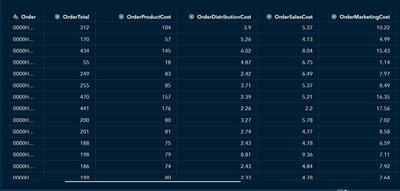Does it help if I provide the source file?

The dataset is very big (total 650mb) but I can extract 10% of it (65mb) for you to test if it helps?

Thank you.

Regards,

KSSam_SAS
SAS Super FREQ

## Re: SASA Viya (Explore & Visualise) - incorrect division answer

Thank you for sharing the screen captures!

It is always a little difficult to process all of the information in a thread like this.

Earlier you said,

The problem I encountered arises when I had to calculate the net profit( which is the calculated item 3) by using OrderTotal less Total Expenses which is made up of 4 cost items (Product, Marketing, Distribution & Sales).

I was able to calculate gross profit without using any aggregate measures; the only difference is that Gross Profit is calculated using OrderTotal Less Product Cost.

When you mention a problem calculating net profit, is this the same issue you were having earlier calculating the percentage? Or is this a different issue?

Multiplication and division calculated items can show confusing results when they are aggregated in a visualization. (Addition and subtraction actually can also give confusing results if there are missing values, because for example 100 + (missing) returns (missing) and that 100 value is not accounted for in the calculated item value.)

If you need to calculate the net profit % in a way that results in a measure instead of an aggregated measure, one workaround would be to pre-calculate the value as part of your data preparation using SAS programming language, SQL syntax, etc.  This example might be helpful?

It is possible that there are other workarounds to try, I am not a great expert in this area.

Sam

## Re: SASA Viya (Explore & Visualise) - incorrect division answer

Hi Sam,

Sorry for the confusion.

Yes, it is the same problem.

I was just trying to simplify the problem by using a generic header (Calculated Item 1,2,3) instead of bogging anyone down with the actual variables (i.e. OrderTotal).

I had to start quoting the actual variable name when we started to talk in detail.

You bought up a very good point about missing data or some incorrect data messing up the calculations.

However, if it is a missing data issue, would you say that it will affect the aggregated measure as well?

I am just a beginner using SAS Viya and I am very thankful for your help and inputs. 🙂

I will take a look at the example but I am not very confident of doing coding in SAS (or anywhere) though.Sam_SAS
SAS Super FREQ

## Re: SASA Viya (Explore & Visualise) - incorrect division answer

I believe that the aggregated measure should not be affected by the missing value issue, at least for Sum aggregation.

The missing value problem can be handled in different ways. You could filter your objects or even the data source to hide the missing values. You could add IF/ELSE logic to your calculated items that replaces missing values with zeroes. You could just add a Text object to your report that explains the issue. For a scientific or academic audience, working around / hiding the missing values might not be acceptable.

Besides calculating your percent of total in the data preparation stage, you could create an aggregated data source as a workaround. Aggregated data sources use extra memory and cause extra processing, so they are not recommended for large data and for production environments.

Sam

Discussion stats
• 7 replies
• 274 views
• 0 likes
• 2 in conversation